# Ordinal Logistic Regression using SPSS

## What is it All About?

Ordinal Logistic regression analysis is a method to determine the reason-result relationship of independent variable(s) with an Ordinal dependent variable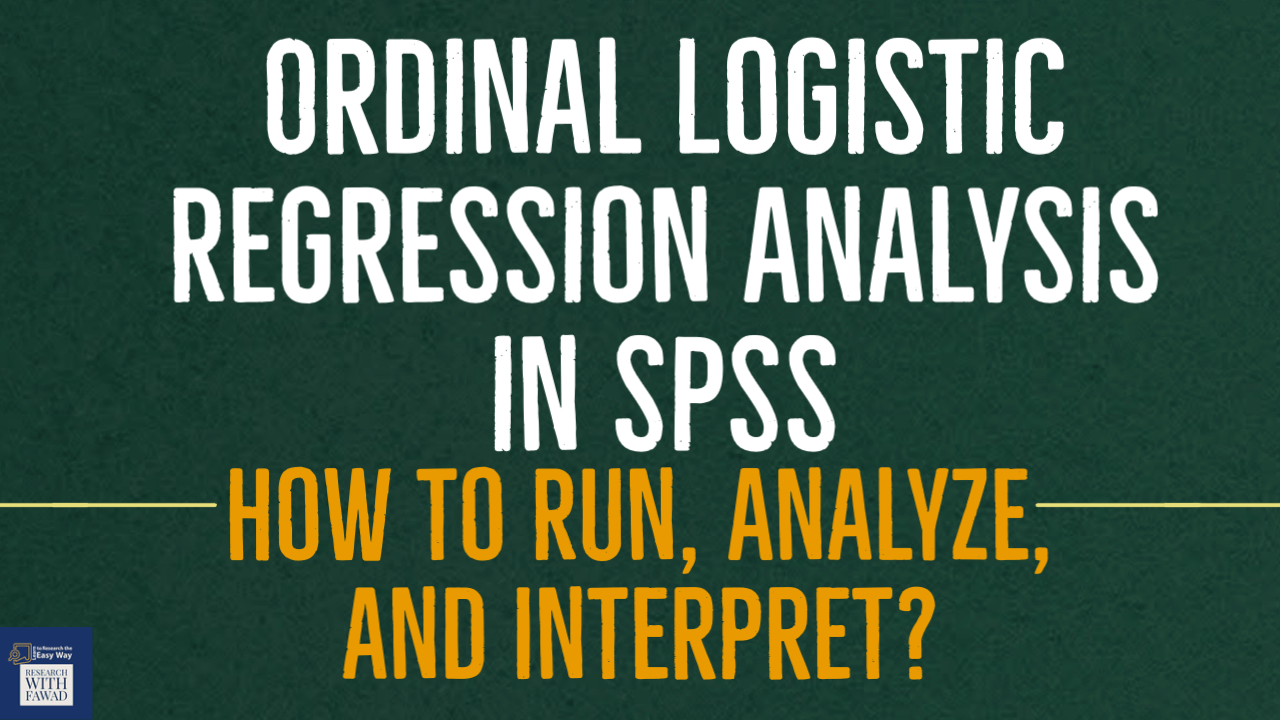### Learn to Analyze Data using Ordinal Logistic Regression using SPSS

The tutorial is a step by step guide on how to perform Ordinal Logistic Regression using SPSS.

## The Concept of Ordinal Logistic Regression

#### "What to do when we have a Likert Scale outcome variable in Research"

Regression technique is used to assess the strength of a relationship between one dependent and independent variable(s). It helps in predicting value of a dependent variable from one or more independent variable.

Linear regression analysis requires the outcome/criterion variable to be measured as a continuous variable. However, there may be situations when the researcher would like to predict an outcome that is Ordinal (for instance Strongly Disagree to Strongly Agree).

In such situation, a scholar can use Ordinal Logistic Regression to assess the impact of one of more predictor variables on the outcomes. Ordinal Logistic regression analysis is a method to determine the reason-result relationship of independent variable(s) with dependent variable

## Examples of Ordinal Logistic Regression

#### A scholar may utilize Ordinal logistic regression in following situations

• A restaurant would like to assess factors that lead to higher customer satisfaction ratings (Strongly Dis-satisfied (1) to Strongly Satisfied(5)).
• A college would like to assess student confidence level (Low (1) to High (5)) of a student based on Age, Grade, Aptitude Test Results.
• A HR researcher would like to ascertain how factors like experience, years of education, previous salary, university ranking affect the selection chances of a candidate in a job interview (Low (1) to High (5).
• A scholar would like to predict interest of University Students in their studies (Low (1) to High (5)) based on independent variables that include Assignments, Co-curricular activities, Gender, and Age.

## Assumptions

• Logistic regression does not assume a linear relationship between the dependent and independent variables.
• The independent variables need not be interval, nor normally distributed, nor linearly related, nor of equal variance within each group
• Homoscedasticity is not required. The error terms (residuals) do not need to be normally distributed.
• The dependent variable in logistic regression is not measured on an interval or ratio scale. The dependent variable must be a dichotomous ( 2 categories) for the binary logistic regression.
• The categories (groups) as a dependent variable must be mutually exclusive and exhaustive; a case can only be in one group and every case must be a member of one of the groups.
• Larger samples are needed than for linear regression because maximum coefficients using a ML method are large sample estimates. A minimum of 50 cases per predictor is recommended (Field, 2013)
• Hosmer, Lemeshow, and Sturdivant (2013) suggest a minimum sample of 10 observations per independent variable in the model, but caution that 20 observations per variable should be sought if possible.
• Leblanc and Fitzgerald (2000) suggest a minimum of 30 observations per independent variable.

## Example Problem

For the purpose of this tutorial, i am considering the following example.

• A scholar would like to predict interest of University Students in their studies (Low (1) to High (5)) based on independent variables that include Assignments, Co-curricular activities, Gender, and Age.

## How to Run Ordinal Logistic Regression

Step 1: In SPSS, Go to Analyze -> Regression -> Ordinal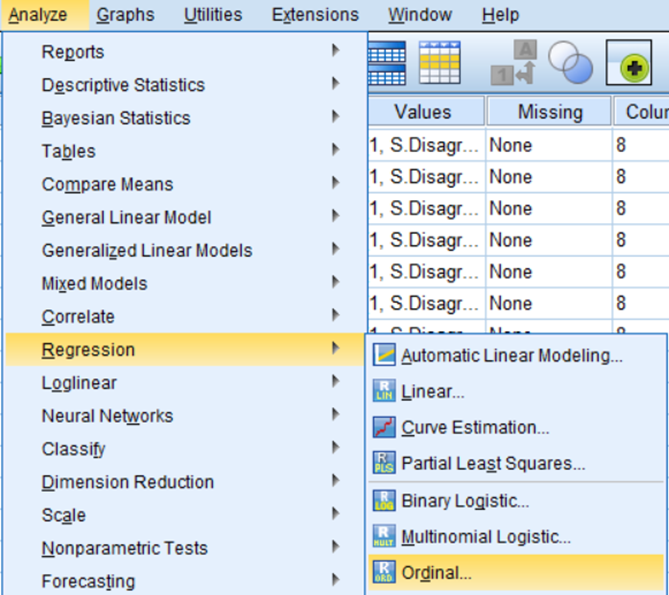Step 2: Next, The Ordinal Logistic Regression Dialog Box will Appear. Add Interest in the Dependent Box and Add IVs, Assignments, CCA, and Gender in the Factor(s) List Box and Age in the Covariates list box.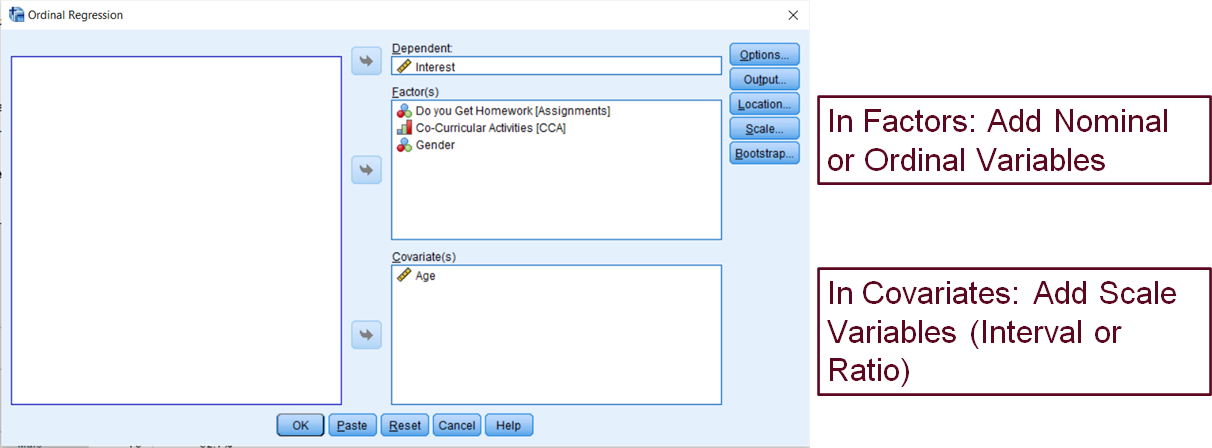Step 3: Next, Select Options, make sure Logit is selected from Link combo box.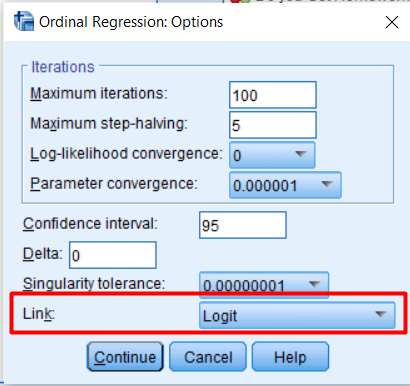Step 4: Press, Output, and Select Test of Parallel Lines.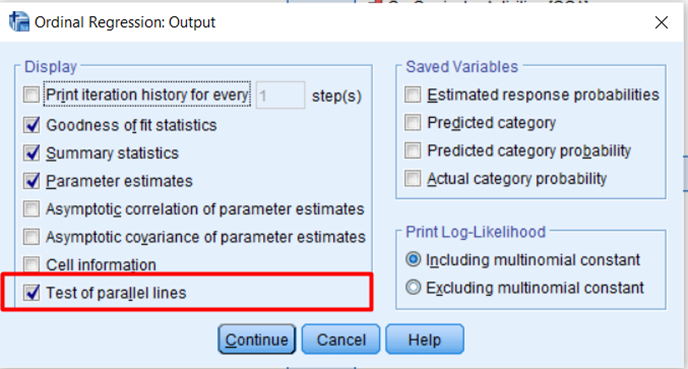## Interpreting Ordinal Logistic Regression

### Case Processing Summary and Encoding

The first section of the output shows Case Processing Summary highlighting the cases included in the analysis. In this example we have a total of 144 respondents.